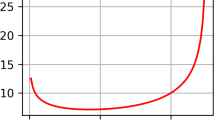# Energy Landscape of the Two-Component Curie–Weiss–Potts Model with Three Spins

## Abstract

In this paper, we investigate the energy landscape of the two-component spin systems, known as the Curie–Weiss–Potts model, which is a generalization of the Curie–Weiss model consisting of $$q\ge 3$$ spins. In the energy landscape of a multi-component model, the most important element is the relative strength between the inter-component interaction strength and the component-wise interaction strength. If the inter-component interaction is stronger than the component-wise interaction, we can expect all the components to be synchronized in the course of metastable transition. However, if the inter-component interaction is relatively weaker, then the components will be desynchronized in the course of metastable transition. For the two-component Curie–Weiss model, the phase transition from synchronization to desynchronization has been precisely characterized in studies owing to its mean-field nature. The purpose of this paper is to extend this result to the Curie–Weiss–Potts model with three spins. We observe that the nature of the phase transition for the three-spin case is entirely different from the two-spin case of the Curie–Weiss model, and the proof as well as the resulting phase diagram is fundamentally different and exceedingly complicated.

This is a preview of subscription content, log in via an institution to check access.

Price excludes VAT (USA)
Tax calculation will be finalised during checkout.

### Similar content being viewed by othersJungkyoung Lee

### Chaos in the Mixed Even-Spin Models

Wei-Kuo ChenNot applicable.

## Code Availability

Not applicable.

1. For notational simplicity, we do not stress the dependency of this object to N; the same convention will be used throughout this paper.

## References

1. Beffara, V., Duminil-Copin, H.: The self-dual point of the two-dimensional random-cluster model is critical for $$q\ge 1$$. Probab. Theory Relat. Fields 153, 511–542 (2012)

2. Collet, F.: Macroscopic limit of a bipartite Curie-Weiss model: dynamical approach. J. Stat. Phys. 157, 1301–1319 (2014)

3. Costeniuc, M., Ellis, R.S., Touchette, H.: Complete analysis of phase transitions and ensemble equivalence for the Curie-Weiss-Potts model. J. Math. Phys. 46, 063301 (2005)

4. Cuff, P., Ding, J., Louidor, O., Lubetzky, E., Peres, Y., Sly, A.: Glauber dynamics for the mean-field Potts model. J. Stat. Phys. 149, 432–477 (2012)

5. Duminil-Copin, H., Sidoravicius, V., Tassion, V.: Continuity of the phase transition for planar random-cluster and Potts models with $$1\le q\le 4$$. Commun. Math. Phys. (2017)

6. Duminil-Copin, H., Gagnebin, M., Harel, M., Manolescu, I., Tassion, V.: Discontinuity of the phase transition for the planar random-cluster and Potts models with $$q>4$$. arXiv:1611.09877 (2017)

7. Eichelsbacher, P., Martschink, B.: On rates of convergence in the Curie-Weiss-Potts model with an external field. Ann. Henri Poincaré 51, 252–282 (2015)

8. Ellis, R.S., Wang, K.: Limit theorems for the empirical vector of the Curie-Weiss-Potts model. Stoch. Process. Appl. 35, 59–79 (1990)

9. Griffiths, R.B., Pearce, P.A.: Potts model in the many-component limit. J. Phys. A 13, 2143–2148 (1980)

10. Jalowy, J., Löwe, M., Sambale, H.: Fluctuations of the magnetization in the block Potts model. arXiv:2103.16421 (2021)

11. Kesten, H., Schonmann, R.H.: Behavior in large dimensions of the Potts and Heisenberg models. Rev. Math. Phys. 1, 147–182 (1982)

12. Knöpfel, H., Löwe, M., Sambale, H.: Large deviations and a phase transition in the block Potts models. arXiv:2010.15542 (2020)

13. Külske, C., Meißner, D.: Stable and metastable phases for the Curie-Weiss-Potts model in vector-valued fields via singularity theory. J. Stat. Phys. 181, 968–989 (2020)

14. Landim, C., Seo, I.: Metastability of non-reversible, mean-field Potts model with three spins. J. Stat. Phys. 165, 693–726 (2016)

15. Lee, J.: Energy landscape and metastability of Curie–Weiss–Potts model. arXiv:2110.03160 (2021)

16. Liu, Q.: Limit theorems for the bipartite Potts model. J. Stat. Phys. 181, 2071–2093 (2020)

17. Ostilli, M., Mukhamedov, F.: Continuous- and discrete-time Glauber dynamics. First- and second order phase transitions in mean-field Potts models. EPL 101(6), 60008 (2013)

18. Rassoul-Agha, F., Seppäläinen, T.: A Course on Large Deviation with an Introduction to Gibbs Measures, Graduate Studies in Mathematics, vol. 162. American Mathematical Society (2015)

19. Wang, K.: Solutions of the variational problem in the Curie-Weiss-Potts model. Stoch. Process. 50, 245–252 (1994)

20. Wu, F.Y.: The Potts model. Rev. Mod. Phys. 54, 235–268 (1982)

## Funding

This work was supported by Samsung Science and Technology Foundation (Project Number SSTF-BA1901-03).

## Author information

Authors

### Corresponding author

Correspondence to Daecheol Kim.

## Ethics declarations

### Conflict of interest

Not applicable.

Communicated by Eric A. Carle.

### Publisher's Note

Springer Nature remains neutral with regard to jurisdictional claims in published maps and institutional affiliations.

## Appendix A: Proof of Proposition 2.2

### Appendix A: Proof of Proposition 2.2

Here, we present a proof of Proposition 2.2.

### Proof of Proposition 2.2

For $$\varvec{x}\in \Xi _{N}^{2}$$, from the definition of $${\mathbb {H}}_{N}$$,

\begin{aligned}&\sum _{\varvec{\sigma }\in \Omega :\varvec{r}(\varvec{\sigma })=\varvec{x}}\frac{1}{Z_{N,\beta }}e^{-\beta {\mathbb {H}}_{N}(\varvec{\sigma })}, =\frac{N!}{(Nx_{1}^{(1)})!\cdots (Nx_{q}^{(1)})!}\cdot {{\frac{N!}{(Nx_{1}^{(2)})!\cdots (Nx_{q}^{(2)})!}}}\\&\quad \times \frac{1}{Z_{N,\beta }}\exp \left[ {{\frac{\beta }{N(1+J)}}}\left\{ \sum _{k=1,2}\sum _{i=1}^{q}\frac{1}{2}\left\{ (Nx_{i}^{(k)})(Nx_{i}^{(k)}-1)\right\} +J\sum _{i=1}^{q}Nx_{i}^{(1)}Nx_{i}^{(2)}\right\} \right] , \end{aligned}

and by Stirling’s formula, we have

\begin{aligned}&\approx \frac{\exp \left\{ -\beta /(1+J)\right\} }{(\sqrt{2\pi N})^{2(q-1)}\sqrt{\prod _{k=1,2}\prod _{i=1}^{q}x_{i}^{(k)}}Z_{N,\beta }}\\&\qquad \times \exp \left[ N\frac{\beta }{1+J}\left\{ \sum _{k=1,2}\sum _{i=1}^{q}\frac{1}{2}(x_{i}^{(k)})^{2}+J\sum _{i=1}^{q}x_{i}^{(1)}x_{i}^{(2)}-\frac{1+J}{\beta }\left( \sum _{k=1,2}\sum _{i=1}^{q}x_{i}^{(k)}\log x_{i}^{(k)}\right) \right\} \right] ,\\&\quad =\frac{1}{{\widehat{Z}}_{N,\beta ,J}}\exp \left\{ -N\frac{\beta }{1+J}\left( F_{\beta ,J}(\varvec{x})+\frac{1}{N}G_{N,\,\beta }(\varvec{x})\right) \right\} , \end{aligned}

where

\begin{aligned} F_{\beta ,J}(\varvec{x})&=-\sum _{k=1,2}\sum _{i=1}^{q}\frac{1}{2}(x_{i}^{(k)})^{2}-J\sum _{i=1}^{q}x_{i}^{(1)}x_{i}^{(2)}+\frac{1+J}{\beta }\left( \sum _{k=1,2}\sum _{i=1}^{q}x_{i}^{(k)}\log x_{i}^{(k)}\right) ,\\ G_{N,\beta ,J}(\varvec{x})&=\frac{1+J}{2\beta }\log \left( \prod _{k=1,2}\prod _{j=1}^{q}x_{i}^{(k)}\right) +O\left( N^{-(q-1)}\right) . \end{aligned}

We decompose the function $$F_{\beta ,J}$$ into an energy part H and entropy part S. That is,

\begin{aligned} F_{\beta ,J}(\varvec{x})=H(\varvec{x})+\frac{1+J}{\beta }S(\varvec{x}), \end{aligned}

where

\begin{aligned} H(\varvec{x})=-\sum _{k=1,2}\sum _{i=1}^{q}\frac{1}{2}(x_{i}^{(k)})^{2}-J\sum _{i=1}^{q}x_{i}^{(1)}x_{i}^{(2)}\quad \text {and}\quad S(\varvec{x})=\sum _{k=1,2}\sum _{i=1}^{q}x_{i}^{(k)}\log x_{i}^{(k)}. \end{aligned}

$$\square$$

## Rights and permissions

Reprints and Permissions